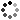# 7th Grade Math Writing Prompts

On Sale
\$5.00
\$5.00
Preview

Get your students writing with these fifty 7th grade math writing prompts. These are designed to prompt thinking and explaining of the common core math concepts. Writing in the math classroom is extremely important and aids to deep understanding and assessment of mathematics. These questions go beyond basic fluency of math concepts. Questions help students analyze and think deeply about the mathematics. As a teacher, you can more easily assess understanding from well-structured questions where students must explain and give examples.

Correlating with the common core, these writing prompts contain the following:
• 8 Number Systems (NS)
• 6 Ratios and Proportions (RP)
• 8 Expressions and Equations (EE)
• 11 Geometry (G)
• 12 Statistics and Probability (SP)
• 5 Mathematical Practices (MP)
These writing prompts come in three different formats:
• Paper strips
• Half sheets of paper with space to respond
These can be used in a variety of ways in the classroom:
• Openers
• Exit slips
• Interactive notebooks
• Homework
• Assessments
• Games
• Centers
The questions are numbered to aid in the process of recording and grading.

Please feel free to contact me with any questions!

Michelle,
Make Sense of Math